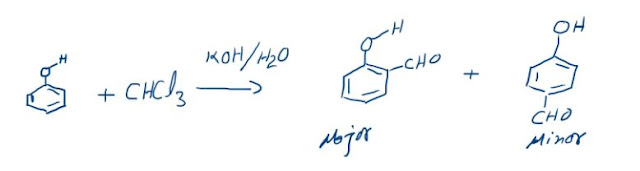## Electrolytic Cell

An electrolytic cell is an arrangement in which electricity is conducted through a solution or a molten salt by the movement of ions. The process of chemical decomposition of the electrolyte by the passage of electricity through its molten or dissolved state is called electrolysis. In electrolysis we supply electrical energy to initiate non–spontaneous processes or we can also say that we convert electrical energy into chemical energy.
In order to pass the current through an electrolytic conductor (aqueous solution or fused electrolyte), two rods or plates (metallic conductors) are always needed which are connected with the terminals of a battery. These rods or plates are known as electrodes. The electrode through which the current enters the electrolytic solution is called the anode (positive electrode) with the electrode through which the current leaves the electrolytic solution is known as cathode (negative electrode).
In the above arrangement the battery supplies electrons to the system. The polarities of the electrodes in this case is opposite of what it was in Galvanic Cell. That is, anode is the positive electrode and the cathode is the negative one. In molten NaCl there are Na+ and Cl ions. Na+ ions move towards the cathode and Cl– ions move towards the anode due to the opposite polarities. Then reduction of cations takes place at the cathode and oxidation of the anions takes place at the anode.

Anode:         2Cl–      Cl2 (g) + 2e–                       Eº = – 1.36 V

Cathode:      2Na+ + 2e–   → 2Na(l)                         Eº = – 2.71 V
Thus, electrolysis of molten NaCl leads to the formation of sodium metal and chlorine gas. The above two processes have no natural tendency to occur but we have made it possible in this case by the supply of external energy.  This movement of ions gives rise to what is known as the electrolytic conduction.
Let us now take a situation where more than one type of cation is present. The ability of cation to move towards the negative electrode and get reduced depends upon the size, mass, positive charge, negative charge etc. It is therefore not possible to predict, qualitatively, the order of reduction of cations, as one factor might enhance it while another factor might hamper it. The only way we can predict this is by giving a quantitative value based on the cumulative effect of all the factors responsible for a cation ability to get reduced. This quantitative value is called the standard reduction potential (SRP). A cation with a higher value of SRP would get reduced in preference to a cation with a lower value of SRP.
For anions the ability to get oxidized is given by the standard oxidation potential which is the reverse of the standard reduction potential of a molecule to form the anion.
For example, consider the following half reactions:
A2+ + 2e   →A                         E = –2 V
B2+ + 2e     B                      E = –1.5 V
C2+ + 2e    C                        E = – 1 V
Amongst the above reactions reduction of A2+ is the most non–spontaneous process and will require the maximum amount of energy to make it happen. Reduction of C2+ ions is the easiest. When these three ions are present discharge of C2+ ions will take place in preference to the other two ions. This phenomenon is called “Preferential discharge”.

### Electrolysis of Sodium Chloride Solution

The solution of sodium chloride besides Na+ and Cl- ions possesses H+ and OH-ions due to ionization of water. However, the number is small as water is a weak electrolyte. When potential difference is established across the two electrodes, Na+ and H+ ions move towards cathode and Cl- and OH- ions move towards anode. At cathode H+ ions are discharged in preference to Na+ ions as the discharge potential of Hions is lower than Na+ ions. Similarly at anode, Cl- ions are discharged in preference to OH- ions.
 At cathode At Anode H+ + e- →  H    2H→  H2 Cl- →  Cl + e- 2Cl →  Cl2
NaClNa+ + Cl-
H2OH+ + OH-

Thus, Na+ and OH- ions remain in solution and the solution when evaporated yields crystals of sodium hydroxide.

### Electrolysis of molten lead bromide.

The reactions occurring at the two electrodes may be shown as follows:
At cathode
Pb2+ (l ) + 2e–   → Pb (l ) Eº = – 0.13 V
(Reduction, primary change)

At anode:
2Br–     → Br2 + 2e– Eº = – 1.09 V
Overall reaction:
PbBr2 (l )          Pb (l )       +             Br2 (g)
At cathode              At anode

### Electrolysis of Copper Sulphate Solution using Platinum Electrodes

CuSO4Cu2+ + SO42-
H2OH+ + OH-
Copper is discharged at cathode as Cu2+ ions have lower discharge potential than H+ ions. OH- ions are discharged at anode as these have lower discharge potential than ions. Thus, copper is deposited at cathode and oxygen gas is evolved at anode
 At cathode At Anode Cu2+ + 2e- → Cu 2OH- → H2O + O + 2e-    O + O → O2

### The Reimer-Tiemann reaction: Reaction and its Mechanism

Reimer-Tiemann Reaction Phenols on reaction with chloroform in the presence of sodium hydroxide (or potassium hydroxide) solution give hydro...